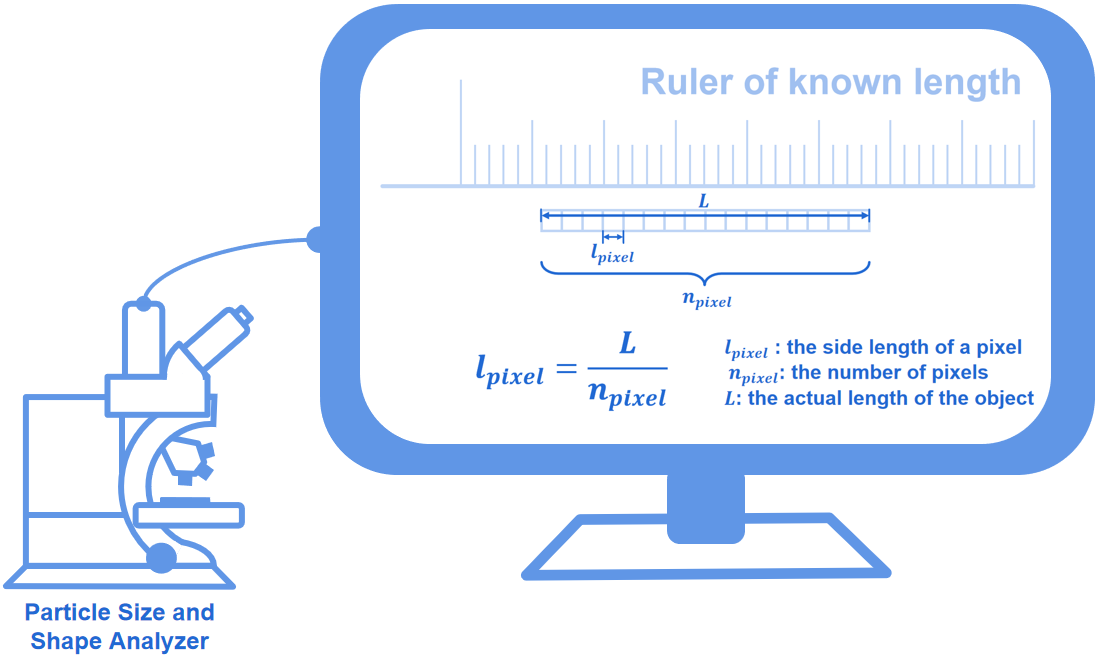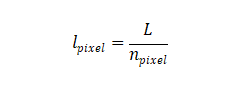Hmoe > Learn > Wiki > What is calibration coefficient?

# What is calibration coefficient?

2023-07-17WIKI

The calibration coefficient refers to the actual length of one of the sides of a single pixel in the image. The area of the pixel can be calculated easily because the pixels are all squares. Particle size and various particle shape parameters are related to length measurement and area calculation. Therefore, the calibration coefficient is of vital importance to particle size and shape analysis.

The general calibration procedure is described below:

• Users need to photograph an object of known length (For example, a ruler) to obtain an image containing the complete object of known length.
• Users need to draw a line segment for the known length and highlight it. The width of the line segment is one pixel.
• The number of pixels on the line segment will be calculated automatically by the software.
• Users need to input the actual length of the line segment to the software. The actual length of one side of a pixel can therefore be obtained; that is, the calibration coefficient.where l pixel is the actual length of a pixel,  L is the actual length of the object and npixel  is the number of pixels.

From the calibration procedure, it is known that the obtained calibration coefficient is only applicable to the photos taken by the same camera under the same magnification. Any operations such as photo distortion, image quality compression, and camera replacement will change the calibration coefficient.Struggling to grasp particle analysis concepts? Our new Beginner's Guide to Particle Analysis is here to help you grasp these concepts in the most accessible manner possible. Download it as a PDF to read on the train, on the plane, or wherever else there’s no internet.